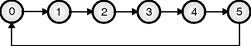Home > VHDL > Counters > Counter examples

1) VHDL Code for 00 to 99 Up Down Counter :

library ieee;

use ieee.std_logic_1164.all;

entity counters is

port (clk : in std_logic;

up_down : in std_logic;

count : out integer range 0 to 127);

end counters;

architecture behav of counters is

begin

process (clk)

variable cnt: integer range 0 to 127;

constant modulus : integer := 99;

begin

if (clk'event and clk = '1') then

if up_down = '1' then

if cnt = modulus then

cnt := 0;

else

cnt := cnt + 1;

end if;

else

if cnt = '0' then

cnt := modulus;

else

cnt:= cnt - 1;

end if;

end if;

end if;

count<= cnt;

end process;

end behav;Fig. 2.13.4 : Mod-6 counter

library ieee;

use ieee.std_logic_1164.all;

entity counter is

port (clear: in bit;

clock: in bit;

count: buffer integer range 0 to 5);

end counter;

architecture example of counter is

begin

process

begin

wait until clock'event and clock = '1';

if (clear = '1' or count >= 5) then

count <= 0;

else

count <= count + 1;

end if;

end process;

end example;

library IEEE;

use IEEE.std_logic_1164.all;

use IEEE.std_logic_unsigned.all;

use IEEE.std_logic_arith.all;

entity count4 is

port ( clk,en,rst: in std_logic;

count: out std_logic_vector(3 downto 0));

end count4;

architecture behav of count4 is

signal cnt: std_logic_vector (3 downto 0);

begin

process (clk,en,cnt,rst)

begin

if (rst='0') then

cnt <= (others =>'0');

elsif (clk'event and clk='1') then

if (en='1') then

cnt <= cnt+'1';

end if;

end if;

end process;

count <= cnt;

end behav;

library IEEE;

use IEEE.std_logic_1164.all;

use IEEE.std_logic_unsigned.all;

entity counter is

port ( CLK : in std_logic;

RST : in std_logic; -- Synchronous reset input RST active high

Q : out std_logic_vector(3 downto 0));

end counter;

architecture counter_arch of counter is

signal TEMP_Q : std_logic_vector(3 downto 0);

begin

process(CLK)

begin

if rising_edge(CLK) then

if RST = '1' then

TEMP_Q <= (others => '0');

else

if (TEMP_Q = "1001") then

TEMP_Q <= (others => '0');

else

TEMP_Q <= TEMP_Q + 1;

end if ;

end if ;

end if;

end process;

Q <= TEMP_Q;

end counter_arch;